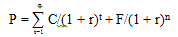## What is Yield to Average Life?

The yield to average life is the yield on a security based on the security's average maturity rather than the maturity date of the issue. The concept is usually applied to bonds with sinking funds, which are often retired early and thus have shorter lives than their maturity dates suggest. The formula for yield to average life is the same as the yield to maturity, with the exception of the use of the average number of years the bond is outstanding rather than the number of years to maturity.

## How Does Yield to Average Life Work?

To understand yield to average life, one must first understand that the price of a bond is equal to the present value of its future cash flows, as shown in the following formula:where:
P = price of the bond
n = number of periods
C = coupon payment
r = required rate of return on this investment
F = maturity value
t = time period when payment is to be received

To calculate the yield to average life, the investor then simply uses trial and error (but more likely a financial calculator or software) to find out what percentage rate (r) will make the present value of the bond’s cash flows equal to today’s selling price. The investor is trying to find out what required rate of return the market is using to calculate the price of the bond.

For example, let's assume you own a 5% Company XYZ bond with a \$1,000 par value that matures in seven years but has an average maturity of three years. If this Company XYZ bond is selling for \$980 today on the market, using the formula above we can calculate that the yield to average life is 2.87%.

Note that because the coupon payments are semiannual, this is the yield to average life for six months. To annualize the rate, we simply use this formula:

Effective Annual Yield = (1 + Periodic Interest Rate)payments per year - 1
= (1 + .0287)2 -1
= 5.82%

## Why Does Yield to Average Life Matter?

The yield-to-average-life calculation is particularly insightful when investors are analyzing bonds with sinking funds (which means that the issuer regularly repurchases or retires a portion of the bonds throughout the life of the issue). In those situations, the bond has a good chance of being retired early, and thus the average life of the bond could be significantly shorter than the stated number of years to maturity. Thus, the yield to average life offers a more realistic indication of what the bond will actually yield, regardless of maturity.

Additionally, bonds with sinking funds that are trading below par will usually trade on a yield-to-average life basis because the ongoing repurchase of the bonds provides some price stability.